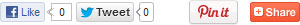# Home

### Write the time as shown in the clocks in the boxes

Page of 39Page of 39Magic SquaresComplete the tableTimeMeasurementsFill in the blanksAdd the followingMoneyFill in the blanks with the words given belowWrite the number of faces, edges and vertices in the following figuresHow many tens and onesWrite the place value of each digit in each numberExpanded formExpanded formSimple multiplicationMultiply the following numbersMultiply these numbersMultiply these numbers and fill the answers in the blanksMultiplication tables write the resultMultiplication tables write the resultMultiplication tables write the resultMultiplication tables write the resultMultiplication and additionRepeated additionSubtract the followingAdditionSubtractionAdditionEven or oddEven and Odd numbersSmallest and highestOrder of NumbersBetween and < or >After and beforeWrite the number names forWrite the number namesMore or lessWrite backward counting from 100 to 1Write counting from 101 to 200